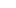## HESI Math Workbook and Practice Questions

### Numbers and Operation

• Decimals, fractions and percent
• Calculate percent increase/decrease
• Solve word problems
• Operations with fractions – add, subtract, divide and multiply
• Estimate solutions
• Solve word problems involving salary and deductions
• Calculate cost of items and tax
• Determine quantities required or cost

How to Study for a Math Test

Basic Math Video Tutorials

Exponents – 8 questions
Basic Math – 10 questions
Basic Math 2 – 10 questions
Word Problems Tutorial and practice
Percent – 18 questions
Metric Conversion – 8 questions
Fractions, Percent and Decimals – 14 questions

### Basic Algebra

• Solve equations with 1 variable
• Perform operations with polynomials – add, subtract, multiple and divide
• Solve inequalities

### Data Interpretation

• Interpret data in graph format
• Measurement
• Convert to and from metric
• Calculate length, weight, height and volume
• Use scale on a map to calculate distances

### Taking the Admissions Assessment? Need to brush up on your math? This is the book for you!

HESI® Math practice questions, easy-to-read tutorials explaining everything in plain language, exam tips and tricks, math shortcuts, and multiple choice strategies! Everything you need, compiled by a dedicated team of experts with everything you need all in one place!

Please note that HESI® is a registered trademark of the Health Education Systems Inc., which was not involved in the production of, and does not endorse, this product.

Here is what the HESI® Math Workbook can do for you:

• Learn then practice your math skills! Practice test questions are the best way to prepare for an exam and this is the book that you need to fully prepare for the HESI math test.
• Practice Tests familiarize you with the exam format and types of questions, giving you more confidence when you take the exam.
• Practice tests are a critical self-assessment tool that reveals your strengths and weaknesses.
• Practice tests allow you to practice your exam time management – a critical exam-writing skill that can easily improve your grade substantially.
• Practice tests reduce Test Anxiety, one of the main reasons for low marks on an exam. Hundreds of questions with detailed solutions and explanations to improve your understand of the basic concepts behind the questions.
• Learn powerful multiple choice strategies designed by exam experts! Includes tips and multiple choice strategies to increase your score you won’t find anywhere else!

Practice Really Does Make Perfect!

The more questions you see, the more likely you are to pass the test. And between our study guide and practice tests, you’ll have over 200 practice questions that cover every category.

Our practice test questions have been developed by our dedicated team of experts. All the material in the study guide, including every practice question, are designed to engage your critical thinking skills needed to pass the test!

Heard it all before?

Maybe you have heard this kind of thing before, and don’t feel you need it. Maybe you are not sure if you are going to buy this book.

Remember though, it only a few percentage points divide the PASS from the FAIL students!

Even if our test tips increase your score by a few percentage points, isn’t that worth it?## Reviews: 5 Stars

Order Now and Start Practicing Today

• Hundreds of Practice Questions
• Multiple Choice Tips and Strategies from Exam Experts
• Self-Assessments to Identify your Strength and Weaknesses
• How to make a Study Plan and Study Schedule
• Practice Test Questions for Basic Math, Fractions, Decimals, Percent, Algebra, Metric Conversion, Word Problems and more
• How to Study for a Math Test
• And a Lot More!HESI Math Workbook Over 200 HESI A2 Math practice questions, plus test tips, how to study math, multiple choice strategies and more!

Pages: 142
Language English
By:

Getting Started
The HESI® Study Plan 9
Making a Study Schedule 12

Estimation
Practice Questions 18
Graphs and Tables
Practice Questions 25

Word Problems
How to Solve Word Problems 32
Types of Word Problems 35

Fractions Decimal & Percent
Fraction Tips, Tricks and Shortcuts 66
Converting Fractions to Decimals 68
Converting Fractions to Percent 69
Decimal Tips, Tricks and Shortcuts 72
Converting Decimals to Fractions 72
Converting Decimals to Percent 72
Percent Tips, Tricks and Shortcuts 73
Converting Percents to Decimals 74
Converting Percents to Fractions 74
Practice Questions 77

Algebra
Solving Algebraic Equations 89
Practice Questions 92

Metric Conversion
Practice Questions 103

Basic Math Multiple Choice

How to Study for a Math Test

How to Prepare for a Test
The Strategy of Studying 120

How to Take a Test
How to Take a Test - The Basics 125
In the Test Room 129
Avoid Anxiety Before a Test 135
Common Test-Taking Mistakes 137

Conclusion

Try a Sample - HESI Math Practice
Written by,

Date Published: Friday, December 16th, 2016
Date Modified: Tuesday, October 6th, 2020